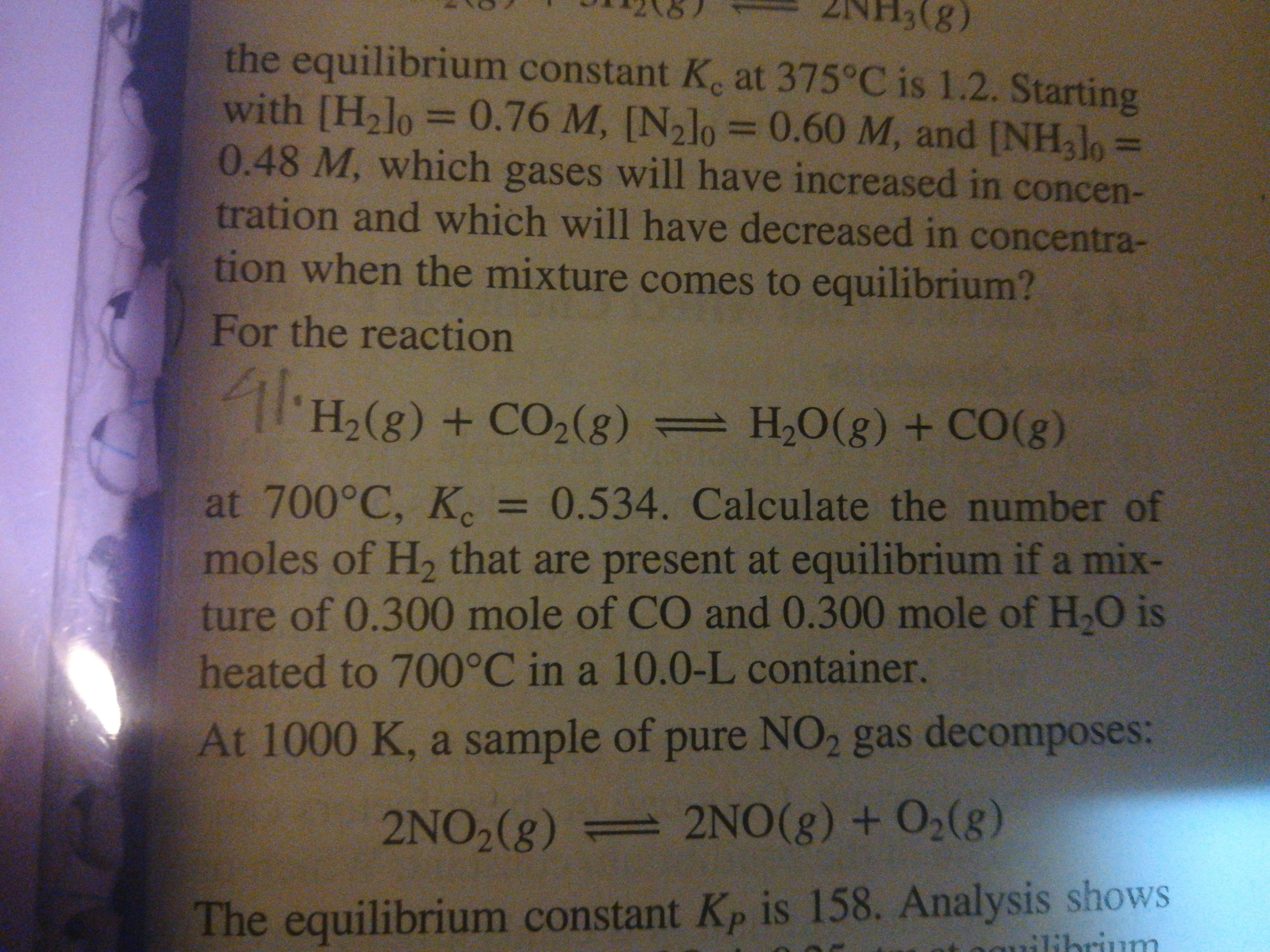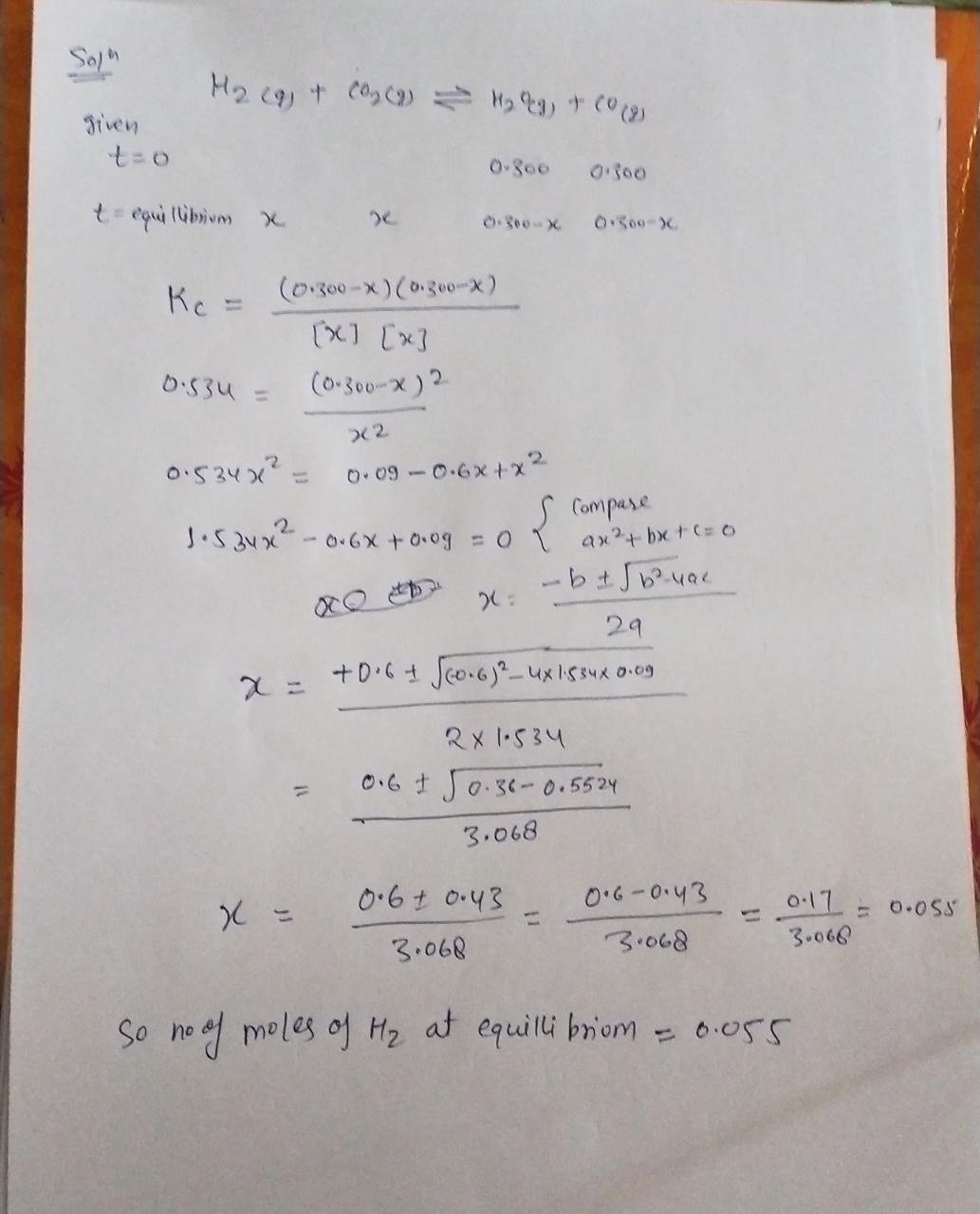# (8):the equilibrium constant K. at 375°C is 1.2. Startingwith [H2lo0.48 M, which gases will have increased in concen-tration and which will have decreased in concentra-tion when the mixture comes to equilibrium?0.76 M, [N,lo D0.60 M, and [NH3J0%3D%3D%3DFor the reaction'H,(g) + CO,(g) = H,O(g) + CO(g)at 700°C, K, =moles of H, that are present at equilibrium if a mix-ture of 0.300 mole of CO and 0.300 mole of H,O is3D0.534. Calculate the number ofheated to 700°C in a 10.0-L container.At 1000 K, a sample of pure NO2 gas decomposes:2NO2(g) 2NO(g) + O2(g)The equilibrium constant Kp is 158. Analysis showsilibrium

Question
18 views

41help_outlineImage Transcriptionclose(8): the equilibrium constant K. at 375°C is 1.2. Starting with [H2lo 0.48 M, which gases will have increased in concen- tration and which will have decreased in concentra- tion when the mixture comes to equilibrium? 0.76 M, [N,lo D0.60 M, and [NH3J0 %3D %3D %3D For the reaction 'H,(g) + CO,(g) = H,O(g) + CO(g) at 700°C, K, = moles of H, that are present at equilibrium if a mix- ture of 0.300 mole of CO and 0.300 mole of H,O is 3D0.534. Calculate the number of heated to 700°C in a 10.0-L container. At 1000 K, a sample of pure NO2 gas decomposes: 2NO2(g) 2NO(g) + O2(g) The equilibrium constant Kp is 158. Analysis shows ilibrium fullscreen
check_circle

Step 1...

### Want to see the full answer?

See Solution

#### Want to see this answer and more?

Solutions are written by subject experts who are available 24/7. Questions are typically answered within 1 hour.*

See Solution
*Response times may vary by subject and question.
Tagged in